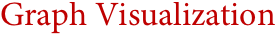Wolfram Archive
Archived 2008. This page no longer reflects the current scope and capabilities of Wolfram's technologies. Find out more about the latest version of Mathematica »

# Graph Visualization(GraphViz, Tom Sawyer, yWorks, ...)
Graph visualization is a mixture of science and art, that has become increasingly important as networks emerge as a key paradigm in many fields. A variety of specialized graph visualization systems have been developed, often available primarily as subroutine libraries. Mathematica is redefining the field of graph visualization, significantly raising the quality and efficiency of automatic graph layout, and allowing immediate graph visualization to become a mainstream part of the everyday computational workflow.
Built into Mathematica is a large collection of original and state-of-the-art graph layout algorithms—developed through a collaboration between algorithm developers and graphic designers at Wolfram Research. Given particular data, Mathematica automatically selects the best algorithms to use, and by being able to draw on Mathematica's vast web of geometric, numeric, graph theoretic, visualization and rendering capabilities, it is able to achieve a very high level of efficiency, routinely handling graphs with even millions of nodes.
A crucial feature is that graph visualization is tightly integrated into the general Mathematica system. Graphs are represented in a general symbolic form that can readily be generated and manipulated by functions in the Mathematica language, or obtained by importing data. Output graphics are also represented symbolically, so that they can immediately be manipulated interactively in arbitrary ways, included in typeset or tabular output, and exported in all standard formats.
Graph Visualization Package Features Built into Mathematica:
Key Advantages of Mathematica for Graph Visualization:
Graph Visualization Package Interoperability:
Interesting Tidbits:
• Mathematica can routinely compute layouts for million-node graphs in under a minute
• The Wolfram Demonstrations Project includes many dynamic graph visualization examples >>
• Mathematica automatically creates graph layouts for almost all sparse matrices in the UFL Sparse Matrix collection >>
See Also Analyses On:
Related Links